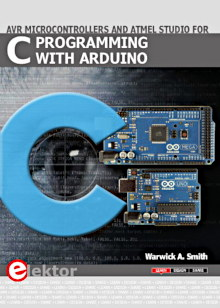Starting Electronics needs your help! Please make a donation to help cover our hosting and other costs. Click the donate button to send a donation of any amount.# Arduino Arrays

Created on: 10 March 2015

Part 17 of the Arduino Programming Course

Arrays are groups of the same kind of data that are placed consecutively in memory. For example, we can have an array of integers (type int) which is two or more integer numbers occurring one after the other.

The key here is that each element in an array is placed directly after the previous element which allows us to access each element in turn using a loop.

An element in an array refers to each value in the array. If we have an array of integers, then each individual integer is referred to as an element of the array. In an array of bytes, each element is a byte (of the Arduino byte type).

## Using Arrays

The sketch below shows the basic use of an array.

```void setup() {
int my_array;     // an array with 5 integer elements
int i;               // used as an index into the array

Serial.begin(9600);

my_array = 23;    // assign a value of 23 to the 1st element
my_array = 1001;  // assign a value of 1001 to the 2nd element, etc.
my_array = 9;
my_array = 1234;
my_array = 987;

// display each number from the array in the serial monitor window
for (i = 0; i < 5; i++) {
Serial.println(my_array[i]);
}
}

void loop() {
}

```

The video below shows the sketch running.

Can't see the video? View on YouTube →

### Defining the Array

In this sketch, an array of 5 elements is defined. This makes space in memory for 5 integers that are put in the memory one after the other. The values that each element contains after the array is defined can contain any random data – whatever happens to be in the memory at the time. It is also possible that the compiler is set to make the values zero, but we can not rely on this.

Defining an array of 5 integers:

```int my_array;     // an array with 5 integer elements
```

In this example the array is of type int, but could be a float, byte, etc.

The array has a name which is my_array in the example.

The array has a length  which means that space for 5 consecutive integers is made in memory.

### Assigning Values to Elements in the Array

Each element is assigned an integer value by referencing it using square brackets [] with the number of the element to access in the brackets.

```my_array = 23;
my_array = 1001;
my_array = 9;
my_array = 1234;
my_array = 987;
```

Note that the element numbering starts from zero  and not one , so the first element in the array is element 0.

In the same way, the last element in the array is numbered one less than the size of the array. In the example, the size of the array is 5, so the number of the last element is 4 – again this is because we are numbering the elements starting with 0.

### Accessing an Array in a Loop

A for loop is used to get the contents of each element in the array in turn and print the values to the Serial Monitor window.

```for (i = 0; i < 5; i++) {
Serial.println(my_array[i]);
}
```

The variable i is used in the for loop as an index into the array to access each element of the array.

In the loop, i is initialized to 0 and then incremented by one each time through the loop so that it counts from 0 to 4. The loop is exited when i becomes 5.

The i variable is used in the array to get the value that the array element is holding starting with element 0 and ending with 4.

```(my_array[i]);
```

In the above code snippet, when i is 0, the first element of the array is accessed and we can then get the value that it contains which is 23 in the example sketch.

Books that may interest you:## More on Initializing Arrays

Instead of initializing each element in the array individually, the array can be defined and initialized in one line as shown in this code.

This sketch does exactly the same as the previous sketch.

```void setup() {
int my_array = {23, 1001, 9, 1234, 987};
int i;

Serial.begin(9600);

// display each number from the array in the serial monitor window
for (i = 0; i < 5; i++) {
Serial.println(my_array[i]);
}
}

void loop() {
}

```

The values to initialize each element with are placed between braces {} after the assignment operator (the equals sign =). The first value between the braces will be assigned to the first element in the array (element number 0), the second number between braces will be assigned to the second element in the array (element number 1), etc.

The code that does the defining and initializing can also be written without the number of elements in the array between the square brackets:

```int my_array[] = {23, 1001, 9, 1234, 987};
```

In this case, the compiler will work out how many elements the array must have based on the number of values that are used to initialize it.

## Uses of Arrays

This part of the course shows that arrays can store data variables of the same type consecutively in memory which allows easy access using a loop.

There are many uses for arrays in programming, for example, arrays can store data that is being logged, such as temperatures. Strings, which are lines of text, are actually arrays as we will see in the next part of this course.

Actual practical uses of arrays will be shown as the course progresses.